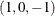### Model Statement Usage

`MODEL` <transform(dependents </ t-options>)><transform(dependents </ t-options>)=> transform(independents </ t-options>)<transform(independents </ t-options>)…> </ a-options> ;

Here are some examples of model statements:

• linear regression

```model identity(y) = identity(x);
```
• a linear model with a nonlinear regression function

```model identity(y) = spline(x / nknots=5);
```
• multiple regression

```model identity(y) = identity(x1-x5);
```
• multiple regression with nonlinear transformations

```model spline(y / nknots=3) = spline(x1-x5 / nknots=3);
```
• multiple regression with nonlinear but monotone transformations

```model mspline(y / nknots=3) = mspline(x1-x5 / nknots=3);
```
• multivariate multiple regression

```model identity(y1-y4) = identity(x1-x5);
```
• canonical correlation

```model identity(y1-y4) = identity(x1-x5) / method=canals;
```
• redundancy analysis

```model identity(y1-y4) = identity(x1-x5) / method=redundancy;
```
• preference mapping, vector model (Carroll, 1972)

```model identity(Attrib1-Attrib3) = identity(Dim1-Dim2);
```
• preference mapping, ideal point model (Carroll, 1972)

```model identity(Attrib1-Attrib3) = point(Dim1-Dim2);
```
• preference mapping, ideal point model, elliptical (Carroll, 1972)

```model identity(Attrib1-Attrib3) = epoint(Dim1-Dim2);
```
• preference mapping, ideal point model, quadratic (Carroll, 1972)

```model identity(Attrib1-Attrib3) = qpoint(Dim1-Dim2);
```
• metric conjoint analysis

```model identity(Subj1-Subj50) = class(a b c d e f / zero=sum);
```
• nonmetric conjoint analysis

```model monotone(Subj1-Subj50) = class(a b c d e f / zero=sum);
```
• main effects, two-way interaction

```model identity(y) = class(a|b);
```
• less-than-full-rank model—main effects and two-way interaction are constrained to sum to zero

```model identity(y) = class(a|b / zero=sum);
```
• main effects and all two-way interactions

```model identity(y) = class(a|b|c@2);
```
• main effects and all two- and three-way interactions

```model identity(y) = class(a|b|c);
```
• main effects and only the `b`*`c` two-way interaction

```model identity(y) = class(a b c b*c);
```
• seven main effects, three two-way interactions

```model identity(y) = class(a b c d e f g a*b a*c a*d);
```
• deviations-from-means (effects or) coding, with an `a` reference level of ’1’ and a `b` reference level of ’2’

```model identity(y) = class(a|b / deviations zero='1' '2');
```
• cell-means coding (implicit intercept)

```model identity(y) = class(a*b / zero=none);
```
• reference cell model

```model identity(y) = class(a|b / zero='1' '1');
```
• reference line with change in line parameters

```model identity(y) = class(a) | identity(x);
```
• reference curve with change in curve parameters

```model identity(y) = class(a) | spline(x);
```
• separate curves and intercepts

```model identity(y) = class(a / zero=none) | spline(x);
```
• quantitative effects with interaction

```model identity(y) = identity(x1 | x2);
```
• separate quantitative effects with interaction within each cell

```model identity(y) = class(a * b / zero=none) | identity(x1 | x2);
```